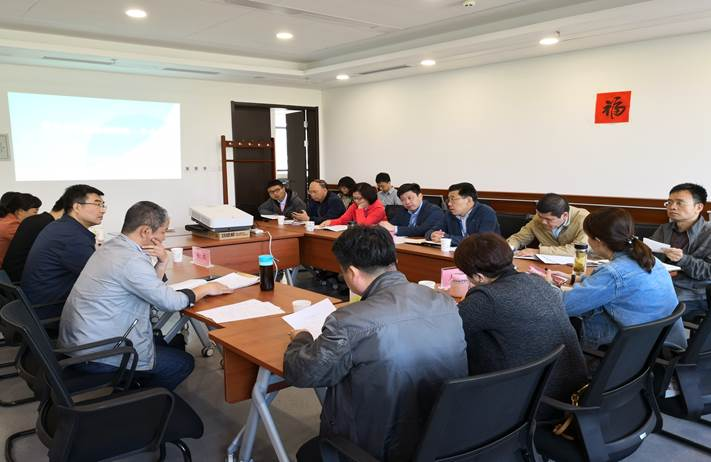• 供应
• 求购
• 企业
• 招商• 西藏
• 陕西
• 甘肃
• 青海
• 宁夏
• 新疆
• 北京
• 天津
• 河北
• 山西
• 内蒙古
• 辽宁
• 吉林
• 黑龙江
• 上海
• 江苏
• 浙江
• 安徽
• 福建
• 江西
• 河南
• 湖北
• 湖南
• 广东
• 广西
• 海南
• 重庆
• 四川
• 贵州
• 云南
• 山东
• 全国

•• 当前位置： 供需平台 >  资讯 >  政策法规 >  积极落实京津冀管廊定额颁发准备工作

积极落实京津冀管廊定额颁发准备工作• 好评统计
• 如果您觉得好，就请您
• 100%(1)
• 差评统计
• 如果您觉得不好，就请您
• 100%(1)
• 资讯导航

•• 热点资讯

•• 西藏
• 陕西
• 甘肃
• 青海
• 宁夏
• 新疆
• 北京
• 天津
• 河北
• 山西
• 内蒙古
• 辽宁
• 吉林
• 黑龙江
• 上海
• 江苏
• 浙江
• 安徽
• 福建
• 江西
• 河南
• 湖北
• 湖南
• 广东
• 广西
• 海南
• 重庆
• 四川
• 贵州
• 云南
• 山东
• 全国